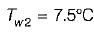Courses

# Basics Of Refrigerating Machine - 2

## 10 Questions MCQ Test Mock Test Series - Mechanical Engineering (ME) for GATE 2020 | Basics Of Refrigerating Machine - 2

Description
This mock test of Basics Of Refrigerating Machine - 2 for Mechanical Engineering helps you for every Mechanical Engineering entrance exam. This contains 10 Multiple Choice Questions for Mechanical Engineering Basics Of Refrigerating Machine - 2 (mcq) to study with solutions a complete question bank. The solved questions answers in this Basics Of Refrigerating Machine - 2 quiz give you a good mix of easy questions and tough questions. Mechanical Engineering students definitely take this Basics Of Refrigerating Machine - 2 exercise for a better result in the exam. You can find other Basics Of Refrigerating Machine - 2 extra questions, long questions & short questions for Mechanical Engineering on EduRev as well by searching above.
QUESTION: 1

### The COP of a heat pump can be increased either by decreasing TH by ΔT or by increasing TL by ΔT. The new COP of the heat pump is

Solution: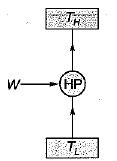Assuming initially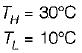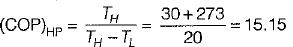If higher temperature is decreased by 5°C. New conditions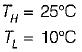Then the COP of heat pump is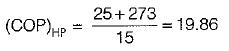If lower temperature is increased by 5°C.
New conditions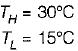Then the COP of heat pump is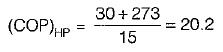Hence COP will be highest when lower temperature is increased rather than decreasing higher temperature by same amount.

QUESTION: 2

### The expression for COP of reversed Brayton cycle is (rp is the pressure ratio)

Solution: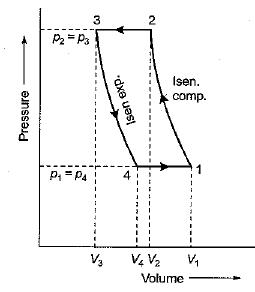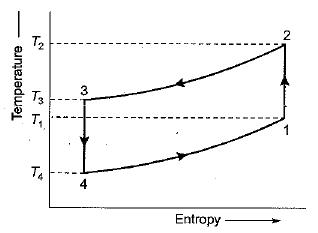Heat absorbed by the air during constant pressure expansion
= cp(T1 - T4)
We know work done during the cycle
= Heat rejected - Heat absorbed
Coefficient of performance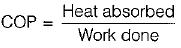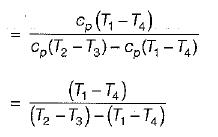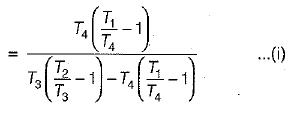We know that for isentropic compression process 1-2.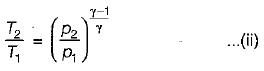Similarly, for isentropic expansion process 3-4.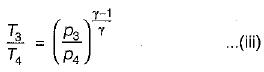Since, p2 = p3 and p1 = p4, therefore from equations (ii) and (iii)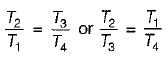Now substituting these values in equation (i), we get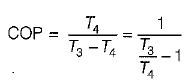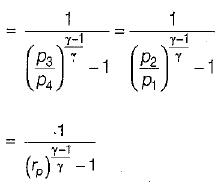QUESTION: 3

### A reversible heat engine runs between high temperature T1 and low temperature T2. The work output of this heat engine is used to run a reversible refrigeration cycle absorbing heat at temperature T3 and rejecting at temperature T2. What is the COP of the combined system?

Solution: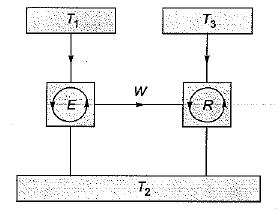COP of the combined system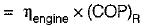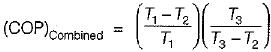QUESTION: 4

If COP of a refrigerator is 5 and efficiency of a heat engine of the same temperature limit is 50%. Then what is the ratio of heat supplied to engine to heat absorbed by the refrigerator from the space

Solution: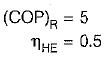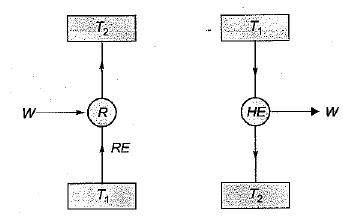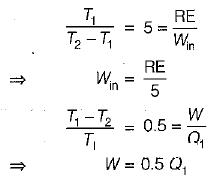By equating, we get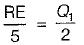⇒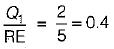QUESTION: 5

COP of a Carnot heat pump operating between -3°C and 27°C is

Solution: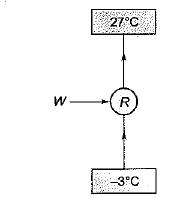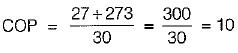QUESTION: 6

A condenser of a refrigeration system rejects heat at a rate of 120 kW, while its compressor consumes a power of 30 kW. The COP of the system would be

Solution: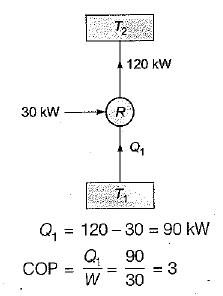QUESTION: 7

If an engine of 40% thermal efficiency drives a refrigerator having a coefficient of performance of 5, then the heat input to engine for each kJ of heat removed from the cold body of the refrigerator is

Solution: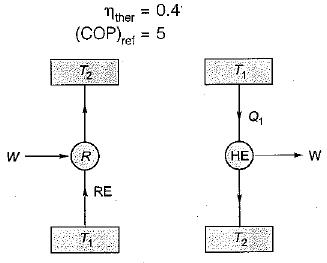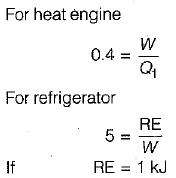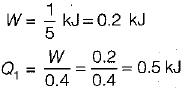QUESTION: 8

In the given figure E is a heat engine with efficiency of 0.4 and R is a refrigerator'given that Q2 + Q4 = 3Q., the COP of the refrigerator is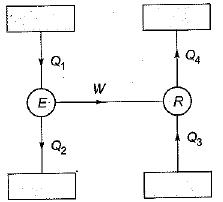Solution: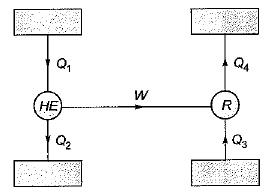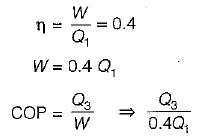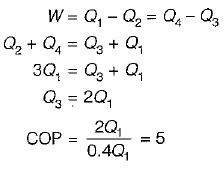QUESTION: 9

A refrigerator working on reversed Car not cycle absorbs 10 kW power and has a COP of 4. What will be the quantity of heat delivered to a building if it works as a heat pump

Solution: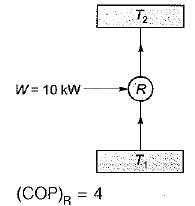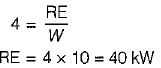Hence heat supplied to building
= 40 + 10 = 50 kW

QUESTION: 10

A water chiller with a capacity of 30 tons of refrigeration cools 20 m3/hr of water entering at 12°C. What is the temperature of water leaving the chiller

Solution: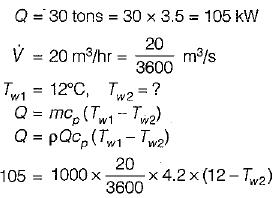or